Anthony Dekker (2007)

Studying Organisational Topology with Simple Computational Models

Journal of Artificial Societies and Social Simulation vol. 10, no. 4 6
<https://www.jasss.org/10/4/6.html>

Received: 28-Feb-2007    Accepted: 25-Jun-2007    Published: 31-Oct-2007###Abstract

The behaviour of many complex systems is influenced by the underlying network topology. In particular, this applies to social systems in which people or organisational units collaboratively solve problems. Network rewiring processes are one useful tool in understanding the relationship between network topology and behaviour. Here we use the Kawachi network rewiring process, together with three simple simulation models of organisational collaboration, to investigate the network characteristics that influence performance. The simulation models are based on the Assignment Problem, the Kuramoto Model from physics, and a novel model of collaborative problem-solving which involves finding numbers with certain characteristics, the existence of which is guaranteed by Lagrange's Theorem. For all three models, performance is best when the underlying organisational network has a low average distance between nodes. In addition, the third model identified long-range connectivity between nodes as an important predictor of performance. The commonly-used clustering coefficient, which is a measure of short-range connectivity, did not affect performance. We would expect that long-range network connectivity would also influence the behaviour of other complex systems displaying global self-synchronization. The paper also demonstrates the utility of simple computational models in studying issues of organisational topology.

Keywords:
Network Rewiring, Small World Networks, Self-Synchronization, Agent Simulation, Collaboration, Problem Solving

###Introduction

1.1
The behaviour of many complex systems, and of organisations in particular, is influenced by their underlying network topology (Albert & Barabási 2002; Barabási 2002; Newman 2003; Watts 2003). Understanding this relationship is important for designing effective organisations to deal with the complexities of the modern world (Moffat 2003). Detailed agent-based simulations (Perez & Batten 2006; Srbljinovic et al. 2003) are a good tool for developing such understanding, but can be time-consuming to develop and run. In addition, it is not always clear which variables are essential to include in such simulations, and adding large amounts of realistic detail can obscure general organisational principles.

1.2
Our interest is in rapidly exploring a wide range of organisational options and variables, with a view to recommending real-world experiments to investigate the most promising alternatives. This can be done using very simple simulation models, each designed to explore from two to four variables and their interactions. Such simulation models would be expressed as between 5 and 20 pages of programming code, not including shared general-purpose libraries.

1.3
In this paper, we describe three simple simulation models, summarised in Figure 1. Each model is intended to simulate aspects of a real network of human beings, carrying out actions in order to achieve individual goals as well as an overall organisational goal. In general, we consider a network of N agents, which may be people or sub-organisations. The first model we describe uses an algorithmic problem, the Assignment Problem, as a proxy for real organisational problems. The second model, the Kuramoto Model, is drawn from physics, and represents the organisation as a network of oscillators. Finally, we introduce a slightly less simple abstraction drawn from mathematics, the Lagrange Model, which offers some additional insights. In particular, it identifies the average connectivity between nodes, which acts as a measure of long-range connectivity, as an important predictor of performance. All three models include abstractions of information transfer, agent actions, and an overall goal, but only the Lagrange Model also models individual agent subgoals.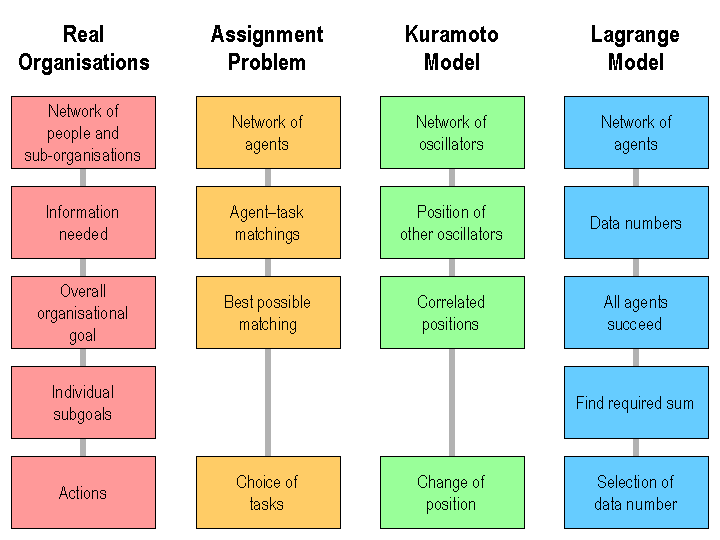Figure 1. Characteristic of the three simple simulation models discussed in this paper, compared to real organisations

###The Kawachi Process

2.1
To understand the relationship between network topology and system behaviour, network rewiring processes, such as those of Watts (2003), and, more recently, Kawachi et al. (2004), are useful. Network rewiring provides a spectrum of different network types which can be provided as input to simulations of complex systems.

2.2
The network rewiring process due to Watts (2003) randomly rearranges links within a network, without altering the number of links. This process creates a spectrum of network types transitioning between regular networks of large diameter, Small-World networks of small diameter, and Random networks, for varying values of the rewiring probability p between 0 and 1.

2.3
The Watts process has been extended by Kawachi et al. (2004) to also transition between Random networks and Scale-Free networks (Albert & Barabási 2002). It thus allows interpolation between four important classes of network, although the Random networks produced by the Kawachi process are not quite true (Erdos-Rényi) random networks. We have improved the Kawachi process to avoid disconnection of the network during rewiring.

2.4
The modified Kawachi rewiring process is controlled by a parameter p between 0 and 5 and takes place in a number of phases (10 phases for our experiment). In each phase, network links x-y are replaced with probability p/10 by links x-z, where the node x has a higher degree than y, and the node z is chosen to be an isolated node from a previous rewiring (if there are any), or chosen randomly (with probability proportional to the degree of nodes plus one) otherwise. For small values of p, this is equivalent to the Watts rewiring process, while for large values of p, the high-degree bias produces networks with Scale-Free characteristics ((Kawachi et al. 2004).

2.5
In our studies, we use an antiprism network with N = 60 nodes as a basis for rewiring (illustrated at the top right of Figure 2). Figure 2 also shows the mean values of the clustering coefficient C (Watts 2003) and the average network distance D between nodes as p is varied from 0 to 5 (the average network distance D between nodes is similar to the "characteristic path length" some authors use). In Figure 2, the average network distance D between nodes decreases sharply as p increases. Between 0.05 and 0.1, Small-World networks are produced. The clustering coefficient C then decreases sharply, reaching a minimum at p = 1. At this point, the networks are similar to, but slightly more structured than, true (Erdos-Rényi) random networks, which are indicated by a circle on the left for comparison. For p > 1, the clustering coefficient C increases slightly, as Scale-Free networks are produced. For larger networks, the curve will be steeper.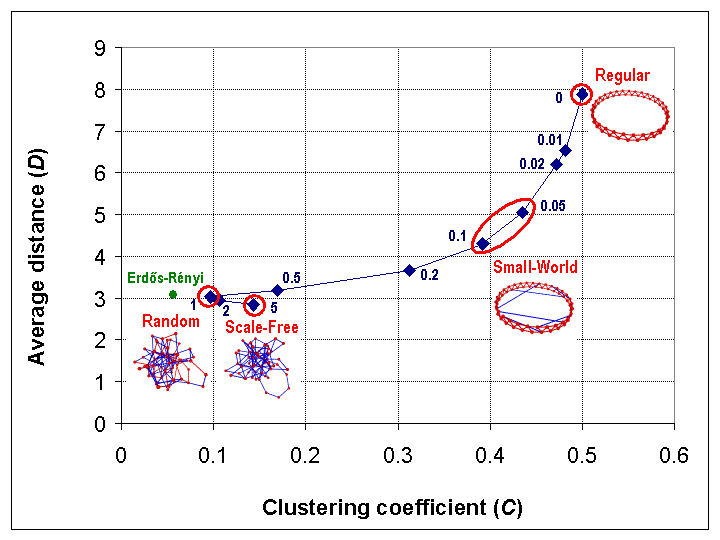Figure 2. Illustration of the Kawachi process, starting with a 60-node antiprism (top right)

2.6
In Figure 2, it can be seen that the average network distance D for both Random and Scale-Free networks is approximately equal to the predicted diameter value for sparse Random or Scale-Free networks of log N / log log N = 2.9 (Bollobás 2001; Chung & Lu 2001). The decrease in D from the initial value is, on average, 14% for the first randomly rewired link (for much larger initial networks, this increases to a limit of 16.6%). Each additional randomly rewired link has a smaller effect, and the decrease in the average network distance D for p > 0 can be approximated by the power law:

 D = 2.04 + 1.07 p-0.32 (1)

Mathematically, the Kawachi process defines a continuous mapping from the interval [0,5] to the set of probability distributions on networks, and hence induces continuous mappings from the interval [0,5] to various network metrics. Figure 2 can be viewed as illustrating the mappings from the interval [0,5] to the metrics C and D.

2.7
We have implemented the Kawachi process as part of a network manipulation library which we have used in the three simulations described in this paper.

###The Assignment Problem

3.1
The first simple computational model that we consider is a network of agents attempting to solve the Assignment Problem (Christofides 1975). Corresponding to the N agents there are N tasks, with a random matrix C(i, j) of costs for each possible agent/task matching. The overall goal is to find a matching of agents i to tasks ti which matches each task to exactly one agent, and minimises the sum of the costs C(i, ti) for the assigned matchings.

3.2
There are, of course, efficient methods for solving the Assignment Problem (Christofides 1975). However, we are using this problem as a proxy for the much more complex problems which real organisations must deal with. In order to have a nontrivial model, agents are only given limited means for solving the problem, based on a purely local view of solution quality.

3.3
Agents begin with a random matching to tasks, and can only improve the matching by swapping their task with one belonging to an agent to which they have a direct network connection. In particular, they do the swapping using a simulated annealing process (Hecht-Nielsen 1990), which improves the final solution quality by allowing locally poor actions initially.

3.4
The simulation was run for 10,000 timesteps. At each timestep, there were two negotiation phases. In the first phase, each agent proposes a "task swap" with a directly connected agent. This proposal is made if the swap would be "good" according to a time-dependent criterion. The change c in cost for the swap (scaled to the range -1 to +1) is calculated, and if c < 0, the swap is of course proposed. However, if c > 0, i.e. there is an increase in cost, the swap is still proposed with probability e-ck/T, where T is a temperature which decreases exponentially from an initial value of T0, and k/T0 = 0.0001. Hence the probability of cost increases is very high initially but is virtually zero toward the end of the simulation. This simulated annealing reduces the chance of the task swapping process becoming trapped in poor local minima.

3.5
In the second phase of negotiation, agents which have made no conflicting proposal of their own accept one of the proposals which they have received. This simulation was implemented in 14 pages of Java programming code (this includes the required matrix manipulation routines, but not the network library).

3.6
The simulation was repeated for 100 different randomly chosen cost matrices C(i, j), and for each matrix, ten network topologies were generated using the Kawachi process with parameters 0, 0.01, 0.02, 0.05, 0.1, 0.2, 0.5, 1, 2, and 5, giving 1,000 data points in all.

3.7
The performance of the network of agents was measured using a quality percentage, defined to be the ratio Q = 100 C0/C, where C is the total solution cost and C0 is the smallest possible solution cost for the given matrix, determined by traditional methods (Christofides 1975). This quality percentage ranged from 9.6% to 32.8%, with a mean of 18.4%. Performance was significantly better for networks with a small average network distance D, particularly Random and Scale-Free networks. The quality percentages fitted the nonlinear regression equation:

 Q = 12.5 + 85.8/D2 (2)

This regression equation, illustrated in Figure 3, explained 57% of the variance in the data (a correlation of 0.76, statistically significant at better than the 10-100 level, by analysis of variance). Since the average network distances D ranged from 2.6 to 7.9, this regression equation corresponds to the average quality percentages ranging from 14% to 25%. Extrapolating, it suggests that if every agent were directly connected to every other (i.e. D = 1), then the quality percentage would average about 98%.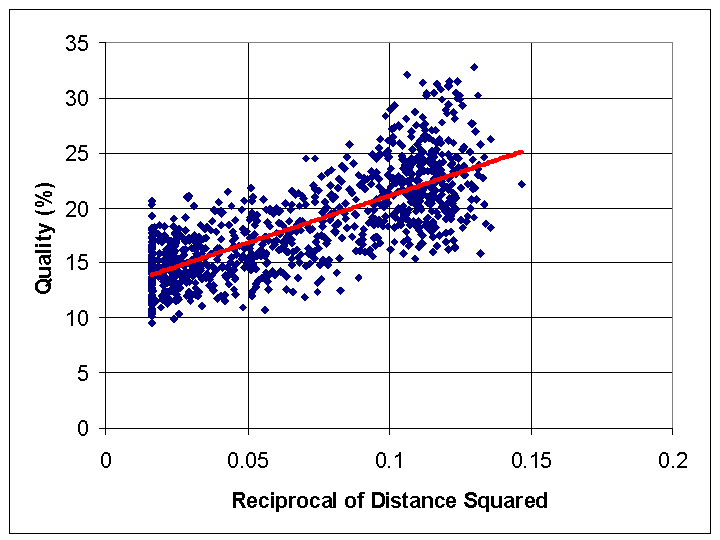Figure 3. Results for Assignment Problem simulation, showing fit of data to the regression equation

3.8
In order to solve the Assignment Problem, agents must be "close to" other agents. If obtaining a good solution requires exchanging tasks between agents which are far apart in the network, there will be a low probability that a good solution will be obtained, since it can only be achieved by sequence of swaps between neighbours. More generally, coordination between agents in a network is facilitated by having a low average network distance D. Similar results are presented for disease transmission by Watts & Strogatz (1998), and by Dekker (2005) for the performance of military agents, although Stocker et al. (2002) do not find a distance effect for their simpler simulation of social agreement.

3.9
For the Assignment Problem, no other network characteristics influence performance (even polynomials in the Kawachi parameter do not explain additional variance). In other words, for this simple problem, having a low average network distance is the only important characteristic of the network topology.

3.10
A low average network distance is in fact characteristic of informal communication networks between people, which have some of the characteristics of Scale-Free networks (Newman 2001; Albert & Barabási 2002; Pujol et al. 2005), although generally with a much higher clustering coefficient, comparable to that of Small-World networks. However, formal organisational hierarchies have a higher average network distance, and consequently may not be the best structures for organisational problem-solving, unless they are supplemented by informal networks (Warne et al. 2005).

###The Kuramoto Model

4.1
A much simpler model of self-synchronization among a network of agents is the networked version of the Kuramoto Model (Strogatz 2000). This model is drawn from physics, and the agents in the network are reduced to simple oscillators, each with a natural frequency fi. The agent actions are reduced to selection of the position or phase of each oscillator, as shown in Figure 4. The agents have no individual or shared goal except for synchronizing the phases of all the oscillators.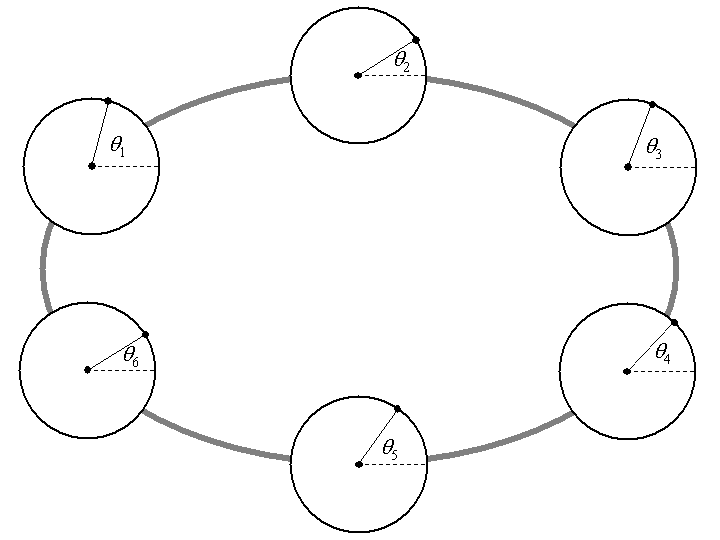Figure 4. Six Kuramoto oscillators connected in a ring network, showing the position (phase) of each oscillator

4.2
The Kuramoto Model has been extensively studied by physicists, but analytical results for finite networks are lacking, especially for networks of arbitrary topology (Strogatz 2000; Dorogovtsev et al. 2007; Kalloniatis 2007). Theoretical analyses of the Kuramoto Model by physicists are thus complemented by simulation studies with varying network topology. The use of the Kawachi process provides an effective way of generating a range of such networks.

4.3
The measure of performance for a network of Kuramoto oscillators is the correlation r between the phases of all the oscillators (Strogatz 2000), which is equal to 1 when the oscillators are synchronized, i.e. all the phases are identical: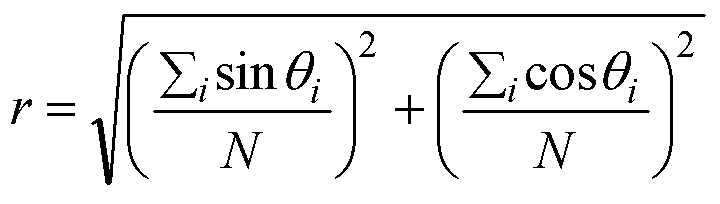(3)

4.4
The agents nudge each other toward synchronization by moving towards the average phase of their neighbours in the network, with the rate of change of each agent's phase being given by: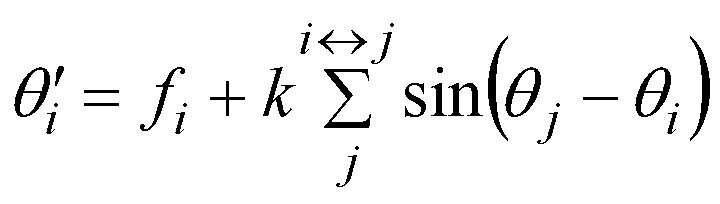(4)

Here the summation is over all agents j directly connected to agent i, and the multiplier k is 0.001 (substantially greater values than this result in chaotic behaviour when discretized). This differential equation can be approximated as a difference equation, specifying the changing phase over one timestep. We iterated this for 100,000 time steps to see if self-synchronization would occur, with the average frequency fi being sufficiently low (around 0.02) to ensure that the timestep behaviour would be a good approximation to the continuous behaviour specified by the differential equation.

4.5
The simulation code for the Kumamoto Model was extremely simple (4 pages of Java code, not including the network library, or 7 pages for a standalone NetLogo version), but gave results quite similar to those of the Assignment Problem. We chose the natural frequencies fi for the oscillators from a uniform distribution in the range 0.02-w/2 to 0.02+w/2 where the width w of the frequency distribution was either 0.01, 0.003, 0.001, 0.0003, or 0.0001. For each frequency distribution width, ten network topologies were generated using the Kawachi process (as for the Assignment Problem), and this was done 50 times, giving 2,500 data points in all. For the largest frequency distribution width (0.01), synchronization was impossible, and the correlation of oscillator phases r averaged 0.23, with only a slight effect due to network topology. For narrower frequency distribution widths (0.001 or less), synchronization became possible, but was achieved only for Random and Scale-Free networks (those with small average network distance), as shown in Figure 5. For these networks, the correlation of oscillator phases r averaged 0.98. Theoretical analysis of the Kuramoto model (Kalloniatis 2007) indicates that the synchronizability limit of 0.001 on the frequency distribution widths should be proportional to the product of the multiplier k and the average network degree.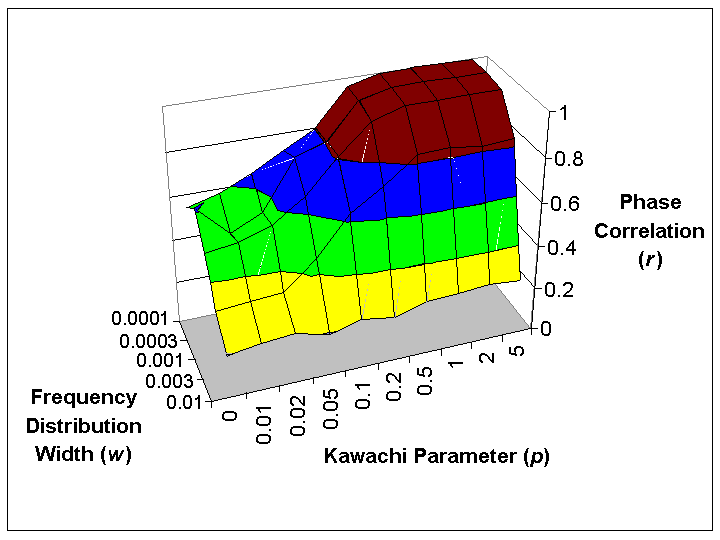Figure 5. Average values of the correlation r between oscillator phases, for different values of the Kawachi parameter used to generate networks and for different frequency distribution widths

4.6
As in the Assignment Problem, good performance required agents to be "close to" the bulk of other agents, i.e. the average network distance D had to be low. Regression analysis of the data required a logarithmic transformation to ensure that the data was approximately normally distributed. The transformed data fitted the regression equation: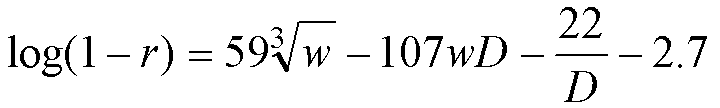(5)

This equation explained 74% of the variance in the data (a correlation of 0.86, with all components statistically significant at better than the 10-100 level). Using a square root rather than a cube root explained only 73% of the variance, and other network characteristics (such as the clustering coefficient, or even polynomials in the Kawachi parameter) did not provide additional explanatory power.

4.7
This equation also emphasises that synchronizability appears to be controlled only by the frequency distribution widths, the average network distance, and the interaction between them. Traditional physics-based approaches tend to approach the Kuramoto Model by calculating a critical value kc for the multiplier which produces synchronization for given networks and values of w (Dorogovtsev et al. 2007). Existing work of this kind does not relate this critical value to attributes of the network (such as average network distance) and hence those results cannot easily be applied to real organisational structures. However, expanding equation (4), it is at least clear that for agents to be "close to" other agents, the average network distance must be small, and must grow at most logarithmically with network size.

4.8
By simulating the Kuramoto Model, we were able to investigate the same basic issue as we did with the Assignment Problem, i.e. self-synchronization within a network of agents; and to obtain similar findings: performance is inversely related to the average network distance D, and so Random and Scale-Free networks perform best. In fact, the simplicity of the Kuramoto Model allows us to study an additional factor: the frequency distribution width, which can be interpreted as the "irrationality" of the agents. As would be expected, agents with high "irrationality" are less able (or, in the extreme case unable) to self-synchronize. The Kuramoto Model thus provides an example where a very simple physics-based model has utility in investigating organisational topologies.

###The Lagrange Model

5.1
We now present a novel model of collaborative problem-solving in organisations, in which a network of agents each begin with a single piece of data. Every agent also has a problem, which is solved when it obtains four pieces of data that "fit together" correctly. Consequently, agents must collaboratively exchange information with each other over the network in order to solve their individual problems.

5.2
We use a very simple instance of this general model, in which each agent begins with a data-number in the range 0,1…59. The problem each agent has is to find four perfect squares which add up to a target-number in the range 33,34…62. By Lagrange's Theorem (Weisstein 2006), this is always possible. Agents are not able to compute data-numbers by subtraction: they can only receive data-numbers, add them together, and compare the totals to their target-number.

5.3
In this model, a data-number is useful if it is a perfect square and either solves the problem, or allows new partial sums less than the target-number to be constructed. For example, an agent with a target-number of 33, which has already received the data-numbers 25 and 0, will find the data-number 4 useful because it solves the problem (25+0+4+4 = 33) or the data-number 1 useful because it creates new partial sums (25+1 = 26, 25+1+1 = 27).

5.4
Agents begin by broadcasting their initial data-numbers to their neighbours. At each timestep following, agents broadcast a randomly chosen data-number from a list of those which they have found useful. However, agents also have an irrationality factor I, similar to the irrationality parameter in the Kuramoto Model, and with probability I, agents may misperceive the usefulness of data-numbers. This will cause them include useless data-numbers (or exclude useful ones) on their list for later re-broadcast. This simulates the irrational behaviour which real human beings in organisational networks will sometimes display.

5.5
The collaboration model above was simulated using network topologies generated by the Kawachi process as in the first two simulations. The model was implemented in 8 pages of Java code. Each of the networks was used as a simulated agent network with irrationality factors I of 0, 0.001, 0.003, 0.01, 0.03, 0.1, and 0.3, and this was done 100 times, giving a total of 7,000 data points. For each of the simulation runs, we recorded an adjusted completion time T, which was the time t, if all agents solved their problems in less than 1000 timesteps, or the product 1000(f + 1), if at 1000 timesteps, f > 0 agents had their problem still unsolved.

5.6
Figure 6 shows the mean values for adjusted completion time T, as a function of p and I. Both the network topology and the irrationality factor I had a significant effect (at better than the 10-100 level) with irrationality causing a substantial increase in the adjusted completion time. Networks intermediate between Small-World and Random networks (specifically, networks with p = 0.5) performed best, with a mean adjusted completion time T of 7,420 (averaged over all values of I).

5.7
The worst case in Figure in 6 is for regular networks with p = 0, I = 0.3, where T = 27,100 (standard deviation 4,400). The best case occurred at p = 0.5, I = 0.001, where T = 5,780 (s.d. 2,400). There was no statistically significant interaction between network topology and the irrationality factor I. These results clarify the preliminary and inconclusive results of a similar previous experiment with a smaller dataset (Dekker 2006).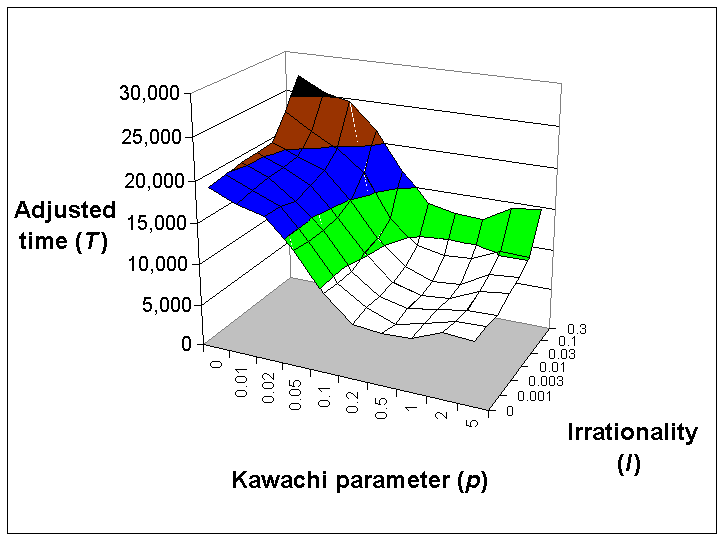Figure 6. Mean adjusted completion time (T) for the Lagrange Model, for different values of the Kawachi parameter p and the irrationality factor I. Networks with p = 0.5 performed best

5.8
As noted in Section 2, the Random networks produced by the Kawachi process are not true (Erdos-Rényi) random networks. Re-running the simulation with true (Erdos-Rényi) random networks gave a mean adjusted completion time T of 7,860 (averaged over all values of I), which was not significantly different from the average of T = 7,750 for the Random networks produced by the Kawachi process at p = 1. However it was slightly worse than the average of T = 7,420 for the p = 0.5 case (significant at the 0.007 level). This demonstrates that the characteristic producing good performance in networks with p = 0.5 is not their similarity to Random networks.

5.9
As in the previous two simulations, reducing the average network distance D between nodes improves performance, but that there is also a structural factor which causes Scale-Free and Random networks to perform less well than networks with p = 0.5. The structural factor is not the clustering coefficient C, although the clustering coefficient has been frequently used in the literature as a measure of network structure (Albert & Barabási 2002; Watts 2003; Kawachi et al. 2004). As a confirmation of this, the experiment was re-run with the network in the lower right of Figure 7: a "Soccer-Ball" network with diagonals through the centre. This network has average network distance D = 3.5 and clustering coefficient C = 0, but performs better than any of the previous networks, with a mean value of T = 3,130 (averaged over all values of I).

5.10
The key to performance in this experiment is long-range connectivity, giving agents multiple pathways for access to distant partners which may have useful information. Studies of real organisations lend support to the importance of such multiple pathways (Warne et al. 2005). Long-range network connectivity can be measured as the average number K of independent network paths between pairs of nodes. By Menger's Theorem (Gibbons 1985), this number of paths can be computed for non-adjacent pairs of nodes using the maximum-flow algorithm, which calculates flows as if the network was composed of pipes. For adjacent nodes, the calculation requires the link between them to be removed, the maximum-flow algorithm applied, and the result incremented by 1. For networks with sufficient symmetry (e.g. the Soccer-Ball network), this calculation gives the vertex connectivity familiar to graph theorists (Gibbons 1985), which is the number of node deletions required to split the network into two components (for both the antiprism and the Soccer-Ball network, this is 4). However, this calculation also provides a good measure of long-range connectivity for nonsymmetrical networks.

5.11
Using the average connectivity K allows us to predict performance moderately well. The average network distance D predicts 57% of the variance in the adjusted completion time T (a correlation of 0.75), but a better predictor is the cubic polynomial:

 T = 22,200 I - 58.9 D3 - 15,700 K + 2,600 K D + 30,500 (6)

This predicts 76% of the variance in the data (a correlation of 0.87). All components of this polynomial are statistically significant at the 10-30 level or better, with the choice of the cubic power for D significant at the 10-5 level, and no other cubic or quartic terms significant.

5.12
Further insight into the results is obtained from Figure 7, which is the analogue of Figure 2 when the clustering coefficient C is replaced by the average connectivity K. Contour lines show values of the cubic polynomial predictor (6). Starting with the 60-node antiprism (top right), performance improves sharply with Small-World networks, and the best adjusted completion time is attained at p = 0.5. For Random networks (p = 1), true (Erdos-Rényi) random networks (shown by a circle for comparison), and Scale-Free networks (p = 5), performance decreases.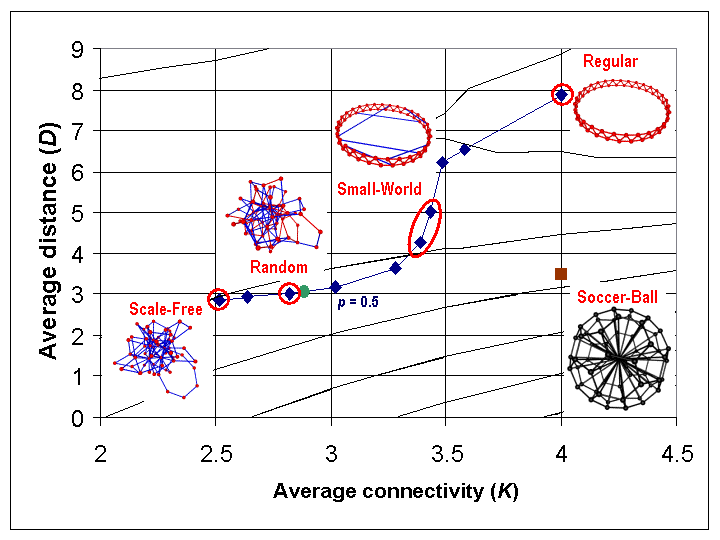Figure 7. Change in average network distance (D) and average connectivity (K) for Kawachi process, overlaid on contour lines for polynomial predictor of the adjusted completion time (T)

5.13
From Figure 7, it can be easily seen why the Kawachi parameter p = 0.5 generates the best-performing network: this case has the best balance between average network distance D and average connectivity K. A low average network distance between nodes reduces the average time to obtain information, while a high average connectivity makes information more accessible. If instead the clustering coefficient C were the key structural factor, we would expect the best-performing networks to be Small-World networks (with p between 0.05 and 0.1). The square at the lower right of Figure 7 shows the values of D = 3.5 and K = 4 for the Soccer-Ball network, and illustrates why this performs even better than the p = 0.5 case: the Soccer-Ball network has a better balance between D and K, since it is even further down the performance slope defined by the contour lines than the p = 0.5 case.

5.14
We would expect that other complex systems displaying global self-synchronization (Moffat 2003) would also have their behaviour determined in part by long-range network connectivity, as measured by the average connectivity K.

###Conclusions

6.1
In this paper, we have demonstrated the utility of simple computational models in studying issues of organisational topology using three simple simulation models. We have combined these models with a modified Kawachi process for rewiring networks, which creates a spectrum of network types transitioning between regular networks of large diameter, Small-World networks of small diameter, Random networks, and Scale-Free networks (Albert & Barabási 2002). The networks produced by the Kawachi process were used as inputs to the three simulation models.

6.2
The first simple computational model we considered was a network of agents attempting to solve the Assignment Problem (Christofides 1975), using "task swapping" combined with simulated annealing (Hecht-Nielsen 1990). Performance was significantly better for networks with a small average network distance D, particularly Random and Scale-Free networks. This finding supports other studies which show the benefits of informal communication networks with low average network distance (Warne et al. 2005), and suggests the use of average network distance as one way of characterising effective organisational structures (Dekker 2007).

6.3
The second model of self-synchronization among a network of agents we considered was the Kuramoto Model (Strogatz 2000). This model is drawn from physics, and the agents in the network are reduced to simple oscillators. Again, performance was significantly better for networks with a small average network distance D, particularly Random and Scale-Free networks. The simplicity of the Kuramoto Model also allowed us to vary the "irrationality" of the agents. As would be expected, agents with high "irrationality" are less able (or, in the extreme case unable) to self-synchronize.

6.4
Finally, we presented a novel model of collaborative problem-solving in organisations, in which agents attempt to find four perfect squares which add up to a given target-number (this can always be done by Lagrange's Theorem). This problem-solving model has since been used for further experiments (Dekker 2007). Performance was best for small average network distance D and low "irrationality," but the Lagrange Model also revealed an additional factor. The best-performing networks were in fact intermediate between Small-World and Random networks, due to a balance between low average network distance and high long-range connectivity. We introduced the concept of average connectivity as a measure of long-range connectivity in the network, defined to be the average number of independent network paths between pairs of nodes. Because human and organisational failings can block certain kinds of information flow, there is a need for independent information channels. This is one reason why informal communication networks complement formal structures (Warne at al. 2005). We would expect that other complex systems displaying global self-synchronization (Moffat 2003) would also have their behaviour determined in part by this long-range network connectivity, and this connectivity measure provides a second way of characterising effective organisational structures.

6.5
Our three simulation experiments demonstrates the useful insights that can be obtained by using relatively simple models of social systems, particularly in combination with network rewiring processes for generating alternative organisational topologies. The Lagrange Model in particular may be useful for other organisational studies.

###Note

The data sets and some software for these experiments are available here.

###References

ALBERT, R and BARABÁSI, A-L (2002), "Statistical mechanics of complex networks." Reviews of Modern Physics, Vol. 74, pp 47-97.

BARABÁSI, A-L (2002), Linked: The New Science of Networks. Cambridge, MA: Perseus Publishing.

BOLLOBÁS, B (2001), Random Graphs, 2nd edition. Cambridge: Cambridge University Press.

CHRISTOFIDES, N (1975), Graph Theory: An Algorithmic Approach. London: Academic Press.

CHUNG, F and LU, L (2001), "The diameter of sparse random graphs." Advances in Applied Math, Vol. 26, pp 257-279.

DEKKER, AH (2005), "Network Topology and Military Performance." In Zerger, A. and Argent, R.M. (eds), MODSIM 2005 International Congress on Modelling and Simulation, Modelling and Simulation Society of Australia and New Zealand, December, pp 2174-2180, ISBN: 0-9758400-2-9. http://www.mssanz.org.au/modsim05/proceedings/papers/dekker.pdf

DEKKER, AH (2006), "Collaborative Problem-Solving and Network Structure." Presentation to Complex Adaptive Systems and Interacting Agents Workshop, Oxford, September.

DEKKER, AH (2007), "Using Tree Rewiring to Study 'Edge' Organisations for C2." Proceedings of SimTecT 2007, Simulation Industry Association of Australia, June, pp 83-88, ISBN: 0-9775257-2-4.

DOROGOVTSEV SN, GOLTSEV AV, and MENDES JFF (2007), "Critical phenomena in complex networks." arXiv:0705.0010v2 [cond-mat.stat-mech], 1 May.

GIBBONS, A (1985), Algorithmic Graph Theory. Cambridge: Cambridge University Press.

KALLONIATIS, AC (2007), "Self-synchronisation and stability in the Network Kuramoto model," to appear.

KAWACHI Y, MURATA K, YOSHII S and KAKAZU Y (2004), "The structural phase transition among fixed cardinal networks." Proc. 7th Asia-Pacific Conf. on Complex Systems, pp 247-255. Australia: Central Queensland University.

MOFFAT, J (2003), Complexity Theory and Network Centric Warfare. Washington, DC: CCRP Publications. http://www.dodccrp.org/files/Moffat_Complexity.pdf

NEWMAN, MEJ (2001), "The structure of scientific collaboration networks." Proc. Nat. Acad. Sci., Vol. 98, No. 2, pp 404-409.

NEWMAN, MEJ (2003), "The structure and function of complex networks." SIAM Review, Vol. 45, No. 2, pp 167-256.

PEREZ, P and BATTEN, D (2006), Complex Science for a Complex World: Exploring Human Ecosystems with Agents. Canberra: ANU E Press, http://epress.anu.edu.au

PUJOL JM, FLACHE A, DELGADO J and SANGÜESA R (2005), "How Can Social Networks Ever Become Complex? Modelling the Emergence of Complex Networks from Local Social Exchanges." Journal of Artificial Societies and Social Simulation, 8(4)12 https://www.jasss.org/8/4/12.html

SRBLJINOVIC A, PENZAR D, RODIK P and KARDOV K (2003), "An Agent-Based Model of Ethnic Mobilisation." Journal of Artificial Societies and Social Simulation, 6(1)1 https://www.jasss.org/6/1/1.html

STOCKER R, CORNFORTH D and BOSSOMAIER T R J (2002), "Network structures and agreement in social network simulations." Journal of Artificial Societies and Social Simulation, 5(4)3 https://www.jasss.org/5/4/3.html

STROGATZ, SH (2000), "From Kuramoto to Crawford: exploring the onset of synchronization in populations of coupled oscillators." Physica D: Nonlinear Phenomena, Vol. 143, pp 1-20.

WARNE L, BOPPING D, ALI I, HART D and PASCOE C (2005), "The challenge of the seamless force: the role of informal networks in the battlespace." Proc. 10th International Command and Control Research and Technology Symposium. Washington, DC: CCRP Publications. http://www.dodccrp.org/events/10th_ICCRTS/CD/papers/215.pdf

WATTS, D J (2003), Six Degrees: The Science of a Connected Age. London: William Heinemann.

WATTS, D J and STROGATZ, S H (1998), "Collective dynamics of 'small world' networks." Nature, Vol. 393, pp 440-442. http://www.tam.cornell.edu/SS_nature_smallworld.pdf

WEISSTEIN, E (2006), "Lagrange's Four-Square Theorem." http://mathworld.wolfram.com/LagrangesFour-SquareTheorem.html